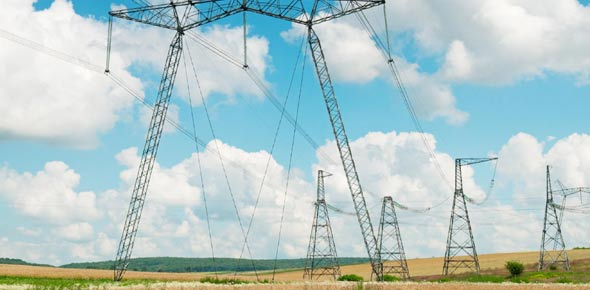# Producing And Measuring Electricity

7 Questions | Total Attempts: 200SettingsMultiple choice revision question on electricity

Related Topics
• 1.
What device do we use to measure the current in a circuit?
• A.

Voltmeter

• B.

Rheostat

• C.

Digital Meter

• D.

Ammeter

• 2.
The measure of the amount of electrical energy that a cell can hold is known as its.................
• A.

Volume

• B.

Capacity

• C.

Time

• D.

Amps

• 3.
In a circuit what particle flow from the negative terminal to the positive terminal?
• A.

Proton

• B.

Neutron

• C.

Electron

• D.

Convectional current

• 4.
A resistor that changes its resistance with changing light intensity
• A.

BBC

• B.

LED

• C.

LDR

• D.

DRL

• 5.
A type of battery that can be use many times.
• A.

Reusuable battery

• B.

Renewable battery

• C.

Rechargeable battery

• D.

Returnable battery

• 6.
An area found around a magnet?
• A.

Line area

• B.

Ground

• C.

Field

• D.

Pasture

• 7.
The size of the induced potential difference in a coil increases if
• A.

The speed of the proton movement increases

• B.

The speed of the proton movement decreases

• C.

The speed of the magnet movement increases

• D.

The speed of the proton movement decreases